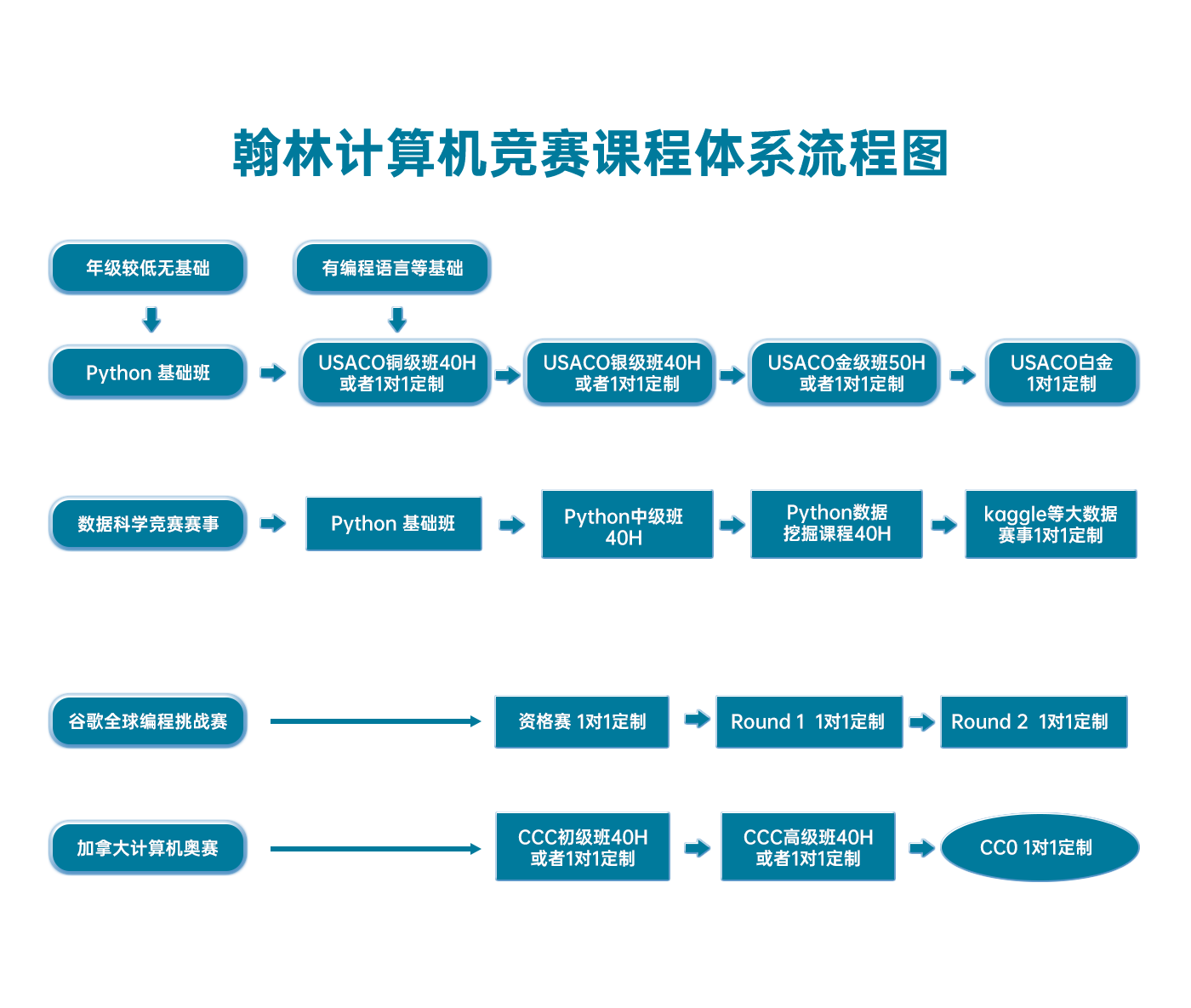# USACO 2015 January Contest, Gold Problem 1. Cow Rectangles

USACO2015JAN-G1

(Analysis by Nick Wu and Brian Dean)

The critical observation for this problem is that an optimal rectangle must have a Holstein on each of its four sides. This motivates the following line-sweep solution:

For every pair of horizontal lines that contains a Holstein, remove all cows that aren't between those two lines. Sweep from left-to-right, keeping track of how many Holsteins have been seen so far without a Guernsey.

If we pre-sort all the cows, then the sweeping process takes linear-time, giving us an O(n3) solution, illustrated in the code below. This was fast enough to obtain full credit for the problem (the problem was intended to the "easier" of the gold problems on this contest).

Faster solutions are possible. Here is a short description of one (of several) ways to achieve an O(n2logn) running time. Recall that the four sides of the optimal rectangle must contain Holsteins; let's denote these by HtHbHl, and Hr, with t meaning "top", b meaning "bottom", l meaning "left" and r meaning "right". We first iterate over all possible choices for HbHb, contributing O(n) to our running time. Having now fixed Hb=(xb,yb), we scan upward (having pre-sorted all points on y), adding all HH's to an STL set, S, keyed on x coordinate. We also keep track of the G with maximum x coordinate less than xb (call it gl) and the G with minimum x coordinate larger than xb (call it gr). We use these values to restrict the entries in S so they belong to the range [gl,gr], by deleting the min or max from SS whenever these fall outside the range. Now for each H we encouter, if it lies in the x range [gl,gr], we test the rectangle with this H as Ht, and with the min and max entries in SS as Hl and Hr. The best rectangle overall is returned. The total scanning time is O(nlog⁡n), for a total running time of O(n2log⁡n).

import java.io.*;
import java.util.*;
public class rectangle {
public static void main(String[] args) throws IOException {
PrintWriter pw = new PrintWriter(new BufferedWriter(new OutputStreamWriter(System.out)));
State[] list = new State[n];
TreeSet<Integer> ys = new TreeSet<Integer>();
for(int a = 0; a < n; a++) {
int x = Integer.parseInt(st.nextToken());
int y = Integer.parseInt(st.nextToken());
list[a] = new State(x, y, st.nextToken().equals("H"));
}
Arrays.sort(list);
ArrayList<Integer> ysArray = new ArrayList<Integer>();
for(int y: ys) {
}
int most = 0;
int area = 0;
for(int i = 0; i < ysArray.size(); i++) {
for(int j = i+1; j < ysArray.size(); j++) {
boolean valid = false;
int lastX = -1;
int now = 0;
for(int a = 0; a < n;) {
int b = a;
int red = 0;
int blue = 0;
while(b < n && list[a].x == list[b].x) {
if(list[b].y >= ysArray.get(i) && list[b].y <= ysArray.get(j)) {
if(list[b].red) {
red++;
}
else {
blue++;
}
}
b++;
}
if(blue > 0) {
valid = false;
now = 0;
}
else if(red + blue > 0) {
if(!valid) {
valid = true;
lastX = list[a].x;
}
now += red;
int currArea = (ysArray.get(j) - ysArray.get(i)) * (list[a].x - lastX);
if(now > most || (now == most && currArea < area)) {
most = now;
area = currArea;
}
}
a = b;
}
}
}
pw.println(most);
pw.println(area);
pw.close();
}
static class State implements Comparable<State> {
public int x,y;
public boolean red;
public State(int a, int b, boolean c) {
x=a;
y=b;
red=c;
}
public int compareTo(State s) {
return x - s.x;
}
}
}

### 翰林USACO课程体系流程图